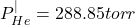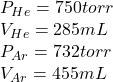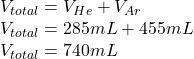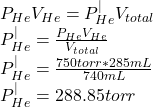A 285-mL flask contains pure helium at a pressure of 750 torr . A second flask with a volume of 455 mL contains pure argon at a pressure of

Question

A 285-mL flask contains pure helium at a pressure of 750 torr . A second flask with a volume of 455 mL contains pure argon at a pressure of 732 torr .If we connect the two flasks through a stopcock and we open the stopcock, what is the partial pressure of helium

in progress 0
5 months 2021-08-29T12:59:22+00:00 2 Answers 0 views 0Explanation:

Given dataRequired

Partial pressure of helium

Solution

First calculate the total volume of gas mixture once the stopcock is opened

SoAs temperature remains constant,by Boyle’s Law we calculate the partial pressure of the helium gas

So2. Explanation:

Below is an attachment containing the solution.